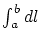Next: The geometry of space Previous: Criticism of the basis of the GRT   Contents

## General remarks

Let us consider the general claims of the GRT. We begin with the myth "on the necessity of the covariance". The unambiguous solution of any differential equation is determined, except the form of the equation, also by specification of the initial and/or boundary conditions. If they are not specified, then, in the general case, the covariance either does not determine anything, or, at changing the character of the solution, can even result in a physical nonsense. If, however, the initial and/or boundary conditions are specified, then with substitution of the solutions we obtain the identities, which will remain to be identities in any case for any correct transformations. Besides, for any solution it is possible to invent the equations, which will be invariant with respect to some specified transformation, if we properly interchange the initial and/or boundary conditions.

The analogies with subspaces are often used in the GRT; for example, a rolled flat sheet is considered. However, the subspace cannot be considered separately from the space as a whole. For example, in rolling a sheet into a cylinder the researcher usually transfers, for convenience, into the cylindrical coordinate system. However, this mathematical manipulation does not influence at all the real three-dimensional space and the real shortest distance.

The simplicity of postulates and their minimum quantity do not still guarantee the correctness of the solution: even the proof of equivalence of GRT solutions is a difficult problem. The number of prerequisites should be, on one hand, sufficient for obtaining a correct unambiguous solution, and, on the other hand, it should provide wide possibilities for choosing mathematical methods of solution and comparison (the mathematics possesses its own laws). The GRT, along with artificial complication of mathematical procedures, has introduced, in fact, the additional number of "hidden fitting parameters" (from metrical tensor components). Since the real field and metrics are unknown in GRT and are subject to determination, the result is simply fitted to necessary one with using a small amount of really various experimental data (first we peeped at the "answer", then we will believe with "a clever air" that it must be in the theory in just the same manner).

Whereas in SRT though an attempt was made to confirm the constancy of light speed experimentally and to prove the equality of intervals theoretically, in GRT even such attempts have not been undertaken. Since in GRT the integralis not meaningful in the general case, since the result can depend on the path of integration, all integral quantities and integral-involving derivations can have no sense.

A lot of questions cause us to doubt as to validity of GRT. If the general covariance of equations is indispensable and unambiguous, then what could be the limiting transition to classical equations, which are not generally covariant? What is the sense of gravitation waves, if the notion of energy and its density is not defined in GRT? Similarly (in the absence of the notion of energy), what is meant in this case by the group velocity of light and by the finiteness of a signal transmission rate?

The extent of the generality of conservation laws does not depend on the method of their derivation (either by means of transformations from the physical laws or from symmetries of the theory). The obtaining of integral quantities and the use of integration over the surface can lead to different results in the case of motion of the surface (for example, the result can depend on the order of limiting transitions). The absence in GRT of the laws of conservation of energy, momentum, angular momentum and center of masses, which have been confirmed by numerous experiments and have "worked" for centuries, cause serious doubts in GRT (following the principle of continuity and eligibility of the progress of science). The GRT, however, has not yet built up a reputation for itself in anything till now, except globalistic claims on the principally unverifiable, by experiments, theory of the evolution of the Universe and some rather doubtful fittings under a scarce experimental base. The following fact causes even more doubt in GRT: for the same system (and only of "insular" type) some similarity of the notion of energy can sometimes be introduced with using Killing's vector. However, only linear coordinates should be used in this case, but not polar ones, for example. The auxiliary mathematical means cannot influence, of course, the essence of the same physical quantity. And, finally, the non-localizability of energy and the possibility of its "spontaneous" non-conservation even in the Universe scales (this is a barefaced "perpetuum mobile") cause us to refuse from GRT completely and either to revise the conception "from zero", or to use some other developing approaches. Now we shall pass from general comments to more specific issues.Next: The geometry of space Previous: Criticism of the basis of the GRT   Contents
Sergey N. Arteha